Examples

Chapter 3 Class 10 Pair of Linear Equations in Two Variables (Term 1)
Serial order wise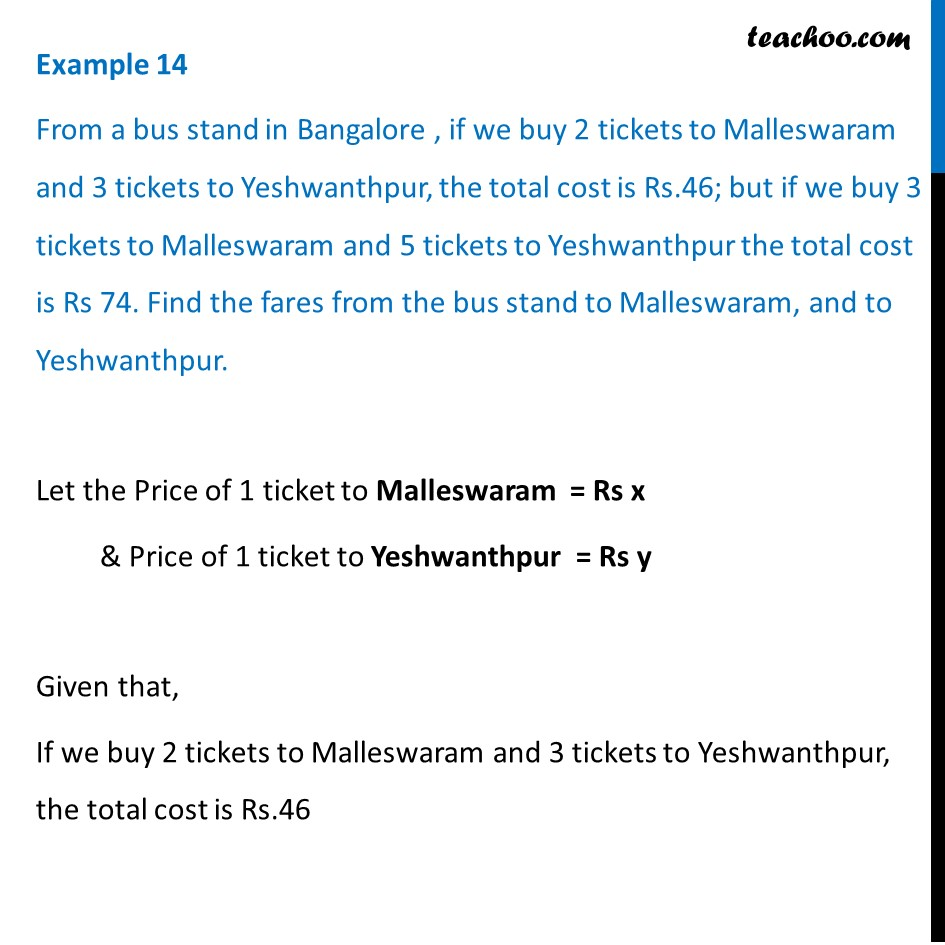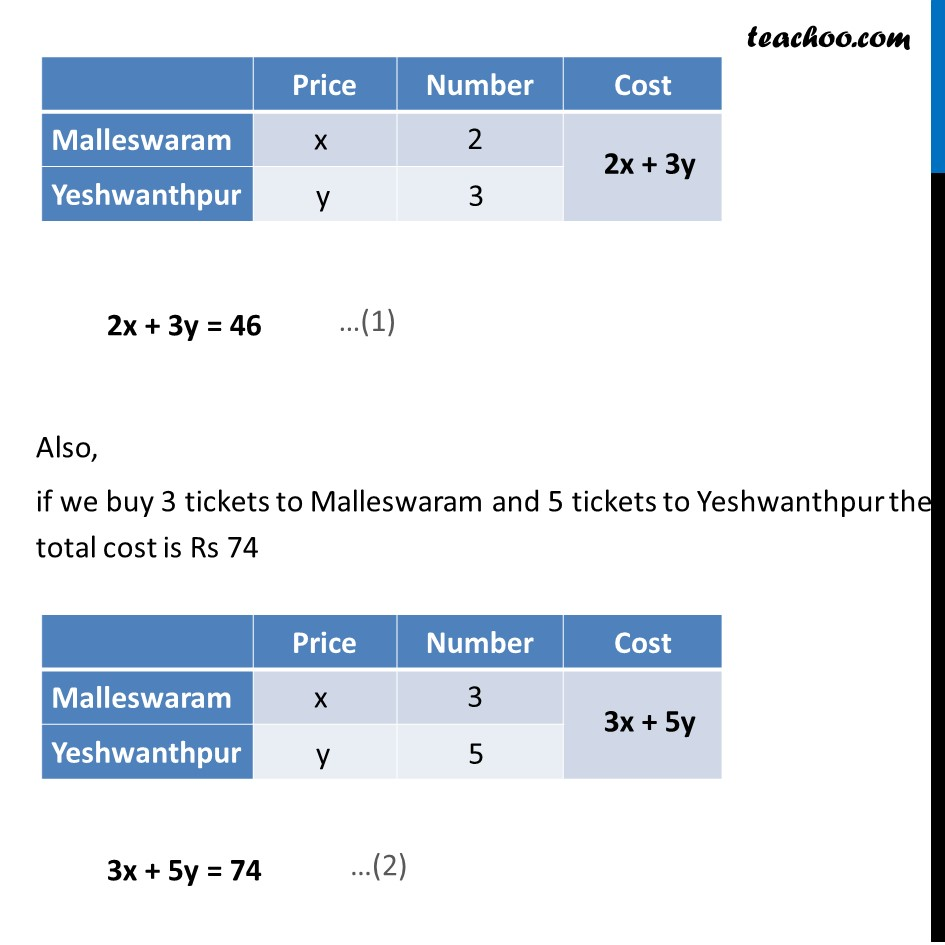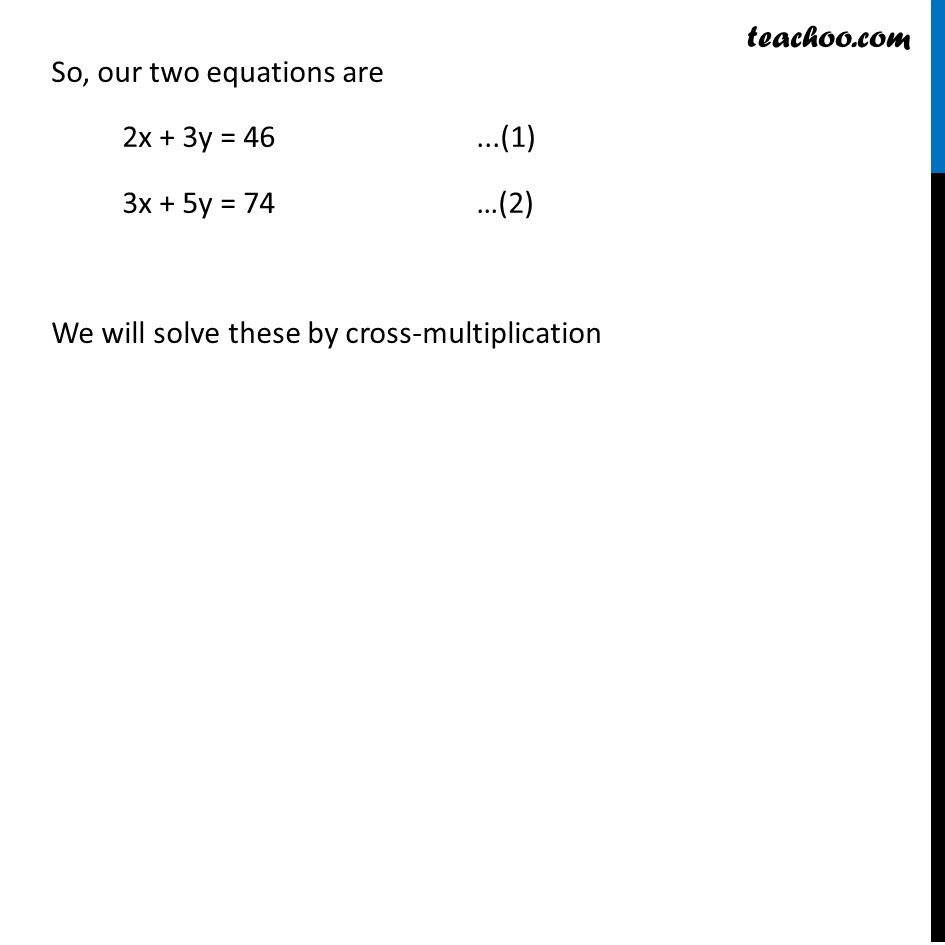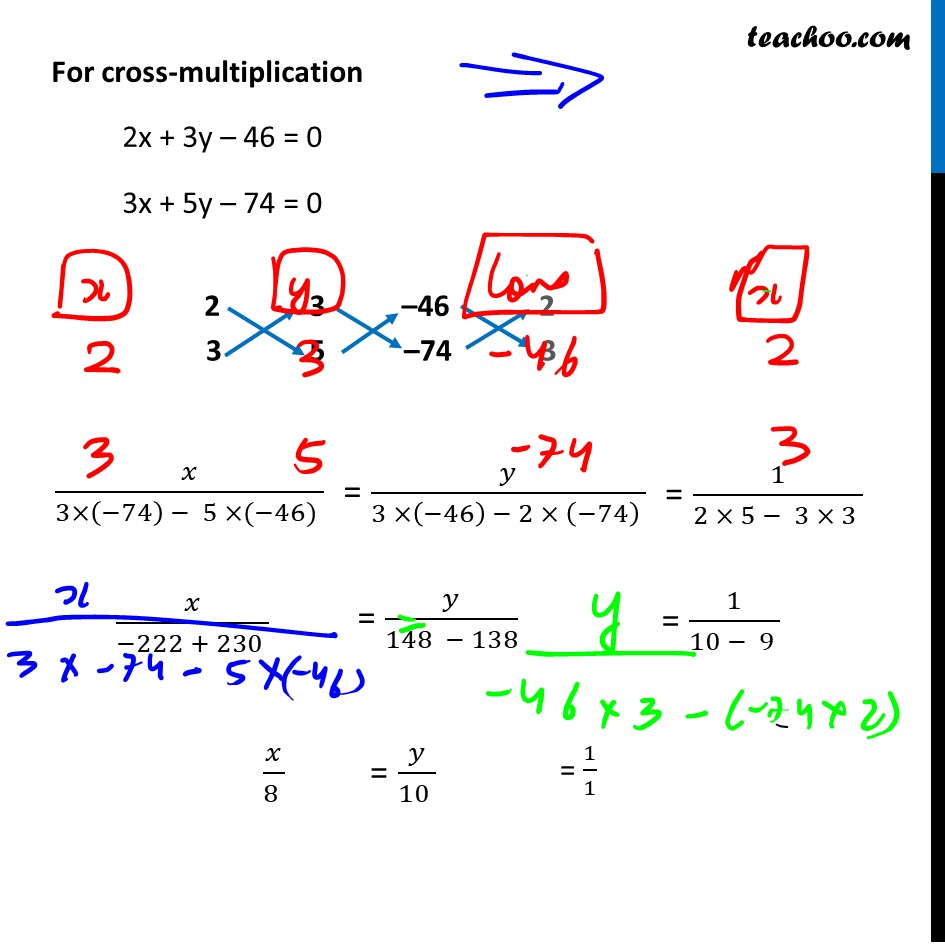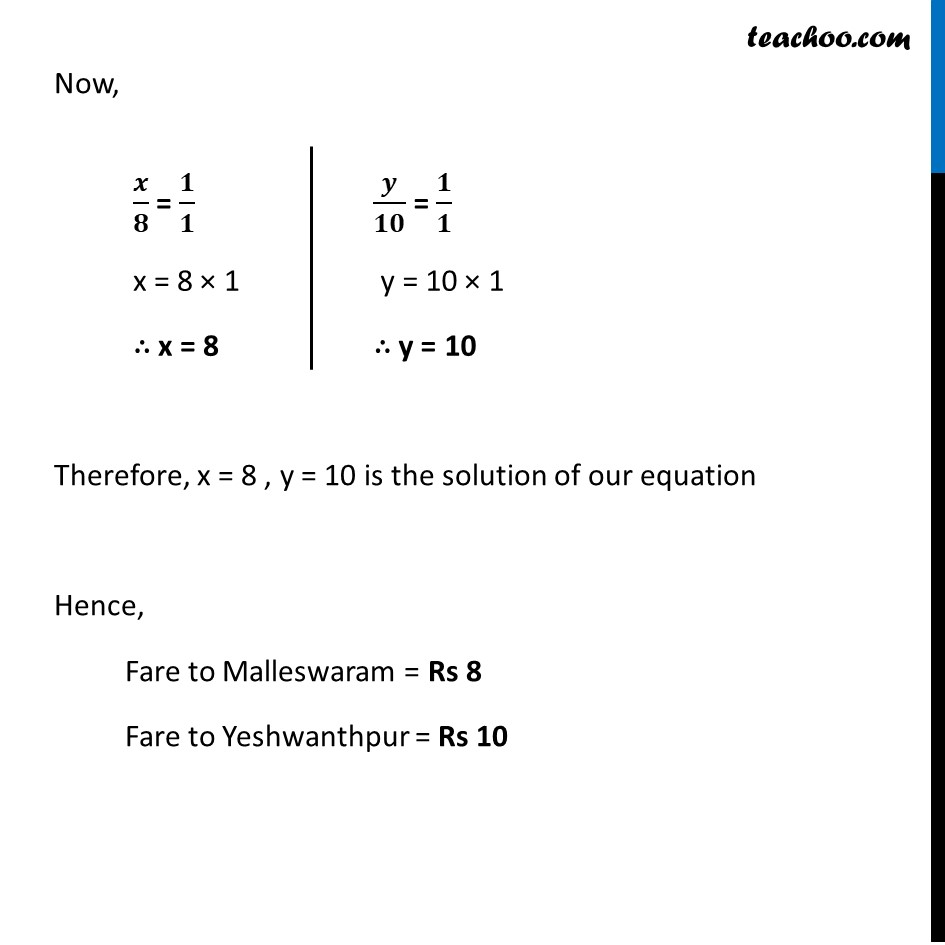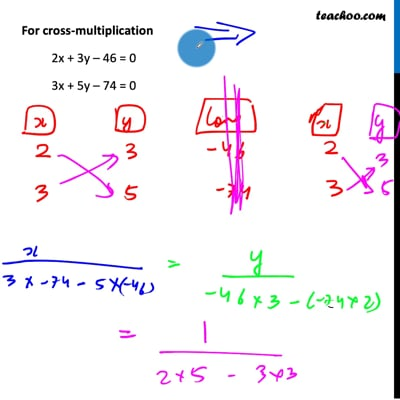This video is only available for Teachoo black users

### Transcript

Example 14 From a bus stand in Bangalore , if we buy 2 tickets to Malleswaram and 3 tickets to Yeshwanthpur, the total cost is Rs.46; but if we buy 3 tickets to Malleswaram and 5 tickets to Yeshwanthpur the total cost is Rs 74. Find the fares from the bus stand to Malleswaram, and to Yeshwanthpur. Let the Price of 1 ticket to Malleswaram = Rs x & Price of 1 ticket to Yeshwanthpur = Rs y Given that, If we buy 2 tickets to Malleswaram and 3 tickets to Yeshwanthpur, the total cost is Rs.46 2x + 3y = 46 Also, if we buy 3 tickets to Malleswaram and 5 tickets to Yeshwanthpur the total cost is Rs 74 3x + 5y = 74 So, our two equations are 2x + 3y = 46 ...(1) 3x + 5y = 74 …(2) We will solve these by cross-multiplication For cross-multiplication 2x + 3y – 46 = 0 3x + 5y – 74 = 0 Now, 𝒙/𝟖 = 𝟏/𝟏 x = 8 × 1 ∴ x = 8 𝒚/𝟏𝟎 = 𝟏/𝟏 y = 10 × 1 ∴ y = 10 Therefore, x = 8 , y = 10 is the solution of our equation Hence, Fare to Malleswaram = Rs 8 Fare to Yeshwanthpur = Rs 10